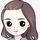cancel
Showing results for
Did you mean:Frequent Visitor

## Cumulative Forecast - S Curve Chart Data

I have followed Sam's tutorial

https://blog.enterprisedna.co/how-to-calculate-a-cumulative-run-rate-in-power-bi-using-dax/

Ideally I need a filter or IF statement where if Actual ISNOTBLANK then Forecast value should equal 0, while still keeping the cumulative sum. Essentiall creating an S-Curve data chart (I have placed the AvgRate and my cumulative measure below the forecast measure)

Essentially what I want to do in power bi is link the actual line and forecast line together, changing table 1 to table 2 (see below tables).

I also have one other query which is to not add the ‘avg daily actual’ to the ‘cumulative forecast’ IF another column, that being ‘Target (hard coded value)’ is equal to zero, changing table 2 to table 3.

Forecast: **bleep** Forecast =

VAR AvgRate = [Avg Rate]

RETURN

CALCULATE(

SUMX(SUMMARIZE(DateTime_Table, DateTime_Table[Date], "Rate", AvgRate), [Rate]),

FILTER(ALLSELECTED(DateTime_Table), DateTime_Table[Date]<=MAX(DateTime_Table[Date])))-AvgRate

Forecast: **bleep** Total Actual = CALCULATE([Actual],DATESMTD(DateTime_Table[Date]),

FILTER (

ALL('Production_Data'[Date and Time]),

'Production_Data'[Date and Time] <= MAX ( 'Production_Data'[Date and Time])

))

Forecast: Avg. Daily Actual =

VAR DaysWithActual = CALCULATE(DISTINCTCOUNT(DateTime_Table[Date]), FILTER(ALLSELECTED(DateTime_Table),[Actual]>0))

VAR CumulativeTotal = CALCULATE([Forecast: **bleep** Total Actual], ALLSELECTED(DateTime_Table[Date]))

RETURN

DIVIDE(CumulativeTotal,DaysWithActual,0)

Thanks

 TABLE 1 Date Daily Actual Actual (cumulitive) Run rate Forecast Target (hard coded value) Target (cumulitive) 12/10/2020 0:00 900 7,425 627 6,897 888 10,688 13/10/2020 0:00 100 7,525 627 7,524 888 11,576 14/10/2020 0:00 627 8,151 888 12,464 15/10/2020 0:00 627 8,778 - 12,464 TABLE 2 12/10/2020 0:00 900 7,425 627 888 10,688 13/10/2020 0:00 100 7,525 627 888 11,576 14/10/2020 0:00 627 8,151 888 12,464 15/10/2020 0:00 627 8,778 - 12,464 TABLE 3 12/10/2020 0:00 900 7,425 627 888 10,688 13/10/2020 0:00 100 7,525 627 888 11,576 14/10/2020 0:00 627 8,151 888 12,464 15/10/2020 0:00 627 8,151 - 12,464 16/10/2020 0:00 627 8,151 - 12,464 17/10/2020 0:00 627 8,151 - 12,464 18/10/2020 0:00 627 8,151 - 12,464 19/10/2020 0:00 627 8,151 - 12,464 20/10/2020 0:00 627 8,151 - 12,464 21/10/2020 0:00 627 8,151 - 12,464 22/10/2020 0:00 627 8,778 888 13,352

2 REPLIES 2Frequent VisitorHi Rena, I have compiled the measures, my problem is I want the forecast measure to be conditional on the target (specifically if the target (grey column) is 0 for the day, do not add the run rate (blue) to the forecast (orange), similar to distinctcount).

Another issue is that it does not start at day 1, see yellow highlighted cell. I have c&ped my measures down the bottom for your ref. any help appreciated.

The picture is a data export of my current measures from power bi.

Actual = CALCULATE(SUM('Actuals'[Actuals A)+SUM(Actuals B]))

Cumulitive Actual = CALCULATE([Actual],DATESMTD(DateTime_Table[Date]),

FILTER (

ALL('Production_Data'[Date and Time]),

'Production_Data'[Date and Time] <= MAX ( 'Production_Data'[Date and Time])

))

Average Daily =

VAR DaysWithActual = CALCULATE(DISTINCTCOUNT(DateTime_Table[Date]), FILTER(ALLSELECTED(DateTime_Table),[Actual)]>0))

VAR CumulativeTotal = CALCULATE([Cumulitive Actual], ALLSELECTED(DateTime_Table[Date]))

RETURN

DIVIDE(CumulativeTotal,DaysWithActual,0)

Target Daily = SUM(Production_Targets[Total Concentrate Target (t/shift)])

Cumulititve Forecast =

VAR AvgRate = [Run Rate]

RETURN

CALCULATE(

SUMX(SUMMARIZE(DateTime_Table, DateTime_Table[Date], "RunRate", AvgRate), [RunRate]),

FILTER(ALLSELECTED(DateTime_Table), DateTime_Table[Date]<=MAX(DateTime_Table[Date])))-AvgRateCommunity Support

1. You want to get two fields: [Forecast] and [Target (cumulitive)]?

2. The calculation logic of these fields as below:

``````Forecast= if ( the value of field [Daily Actual] is not blank, blank(),[Forecast]+[Run rate])
[Target (cumulitive)]=if( [Target (hard coded value)]is blank, not culmulative, cumulitive+Target (hard coded value))``````

3. The first value of [Forecast] 6897 and [Target (cumulitive)] 10688 is default value or the value from the calculation?

If my understanding is correct, here are some similar threads, you can refer them to get it.

Cumulative sum by date by condition

Cumulative sum with condition

Best Regards

Rena

Community Support Team _ Rena
If this post helps, then please consider Accept it as the solution to help the other members find it more quickly.